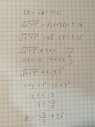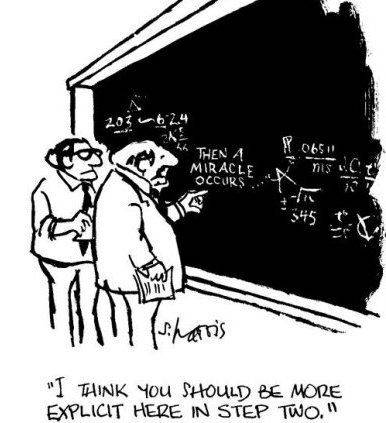# Complex numbers problem |z| - iz = 1-2i

• Callmelucky
In summary: I didn't recognize that. What does that mean, that I have 3 complex numbers in that...It means that when you multiply a complex number by ##i##, you get a different complex number. For example, if ##z = 1 + i##, then ##iz = i + i^2 = i - 1##. So when you have ##z = x + iy## and you multiply it by ##i##, you get ##iz = ix + i^2y = -y + ix##. But keep in mind that ##z## and ##iz## are two different complex numbers.For your problem, you have the equation ##|z| - iz = 1 - 2i##.

#### Callmelucky

Homework Statement
Photo of procedure below.
Relevant Equations
z= x+yi, |z|=sqrt(x^2 + y^2), i^2=-1
Here is my attempt(photo below), but somehow the solution in the textbook is z= 2 - (3/2)i, and mine is z=(-3/2) +2i.

Can someone please tell me where I am making a mistake? I suppose it's something with x being part of the real part of the 1st complex number and x being part of an imaginary number of the 1st complex number. But I don't know how to make it right.

Thank you.

#### Attachments

•WhatsApp Image 2022-12-16 at 20.34.35.jpeg
32.8 KB · Views: 54
Last edited:
Callmelucky said:
Homework Statement:: Photo of procedure below.
Relevant Equations:: z= x+yi, |z|=sqrt(x^2 + y^2), i^2=-1

Here is my attempt(photo below), but somehow the solution in the textbook is z= 2 - (3/2)i, and mine is z=(-2/3) +2i.

Can someone please tell me where I am making a mistake? I suppose it's something with x being part of the real part of the 1st complex number and x being part of an imaginary number of the 1st complex number. But I don't know how to make it right.

Thank you.
Please include the statement of the problem in the text of your post, not merely in the Title.

Also, the image you posted is unreadable or nearly so.

Please learn to use the typing helps at the top of the editing screen, at the very least. Better yet, learn to use LaTeX.

•jim mcnamara
SammyS said:
Please include the statement of the problem in the text of your post, not merely in the Title.

Also, the image you posted is unreadable or nearly so.

Please learn to use the typing helps at the top of the editing screen, at the very least. Better yet, learn to use LaTeX.
I want to learn latex but I don't have time right now. Here is another pic
Here is the new pic(below). I want to learn Latex, but I don't have time rn.

Problem: Solve in the set of complex numbers. |z| -iz = 1-2i

#### Attachments

SammyS said:
Please include the statement of the problem in the text of your post, not merely in the Title.

Also, the image you posted is unreadable or nearly so.

Please learn to use the typing helps at the top of the editing screen, at the very least. Better yet, learn to use LaTeX.
Amen to all of these. As @SammyS noted, the image is pretty much unreadable. However, I was able to work the problem and get the same solution as shown in the book.

Callmelucky said:
I suppose it's something with x being part of the real part of the 1st complex number and x being part of an imaginary number of the 1st complex number.
This is very unclear.
The equation you're working with is ##|z| - iz = 1 - 2i##
Replace z with x + iy and substitute into the given equation. Note that in the complex number x + iy, y is the coefficient of the imaginary part, but both x and y are real numbers.

The new image is slightly more readable, and it looks like you were able to correct your mistake.
Edit: I didn't notice that the real and imaginary parts were reversed.

Last edited:
•ohwilleke
Yeah but I don't understand how is y imaginary part if i is next to x(xi). Shouldn't x be imaginary part?

I know that z= x+yi, but I have xi after multiplying i(x+yi). That is what bothers me.

Callmelucky said:
I know that z= x+yi, but I have xi after multiplying i(x+yi). That is what bothers me.
If ##z = x +yi##, then ##iz = -y +ix## and we see that ##Im(iz) = x##.

OK, so; you said:
1) ##z=x + iy##
2) ## x=2 ##
3) ## y=-\frac{3}{2}##
4) ## z=-\frac{3}{2} + 2i ##

•ohwilleke, FactChecker and PeroK
DaveE said:
OK, so; you said:
1) ##z=x + iy##
2) ## x=2 ##
3) ## y=-\frac{3}{2}##
4) ## z=-\frac{3}{2} + 2i ##

Your only problem is the last line.
But that is not the solution. Solution is z= 2 - (3/2)i

Callmelucky said:
But that is not the solution. Solution is z= 2 - (3/2)i
Reread my post slowly. Do the substitutions in step 4 carefully.

DaveE said:
Reread my post slowly. Do the substitutions in step 4 carefully.
I don't get how did x became y and vice versa

Callmelucky said:
But that is not the solution. Solution is z= 2 - (3/2)i
Let's assume your solution is correct:
$$z = -\frac 3 2 + 2i$$$$\Rightarrow \ |z| = \frac 5 2, iz = -2 - \frac 3 2i$$$$\Rightarrow \ |z| - iz = \frac 9 2 + \frac 3 2 i$$

Last edited:
Callmelucky said:
I don't get how did x became y and vice versa
Me either.••pinball1970, malawi_glenn and jim mcnamara
Callmelucky said:
I don't get how did x became y and vice versa
Do you recognise that ##z## and ##iz## are different complex numbers? In the sense that ##z \ne iz##?

PeroK said:
Do you recognise that ##z## and ##iz## are different complex numbers? In the sense that ##z \ne iz##?
I didn't recognize that. What does that mean, that I have 3 complex numbers in that equation?

Callmelucky said:
I didn't recognize that. What does that mean, that I have 3 complex numbers in that equation?
It means that if ##z = x + iy## then ##iz \ne x + iy##.

•FactChecker
PeroK said:
It means that if ##z = x + iy## then ##iz \ne x + iy##.
I got the right solution. I think I get it now. For some reason I thought that I am not allowed to move stuff from right side to the left. Thank you.

Last edited:
Thanks everyone.

Callmelucky said:
I don't get how did x became y and vice versa
The ##x## and ##y## came from ##z=x+iy## (which you never specifically wrote down). So ##x=2## and ##y = -3/2## means that ##z = 2 - (3/2) i##

Callmelucky said:
Yeah but I don't understand how is y imaginary part if i is next to x(xi). Shouldn't x be imaginary part?
If z = x + iy, then the Re(z) = x and Im(z) = y.
Callmelucky said:
I know that z= x+yi, but I have xi after multiplying i(x+yi).
##iz = i(x + iy) = i^2y + ix = -y + ix##
Re(iz) = -y and Im(iz) = x
The real part is the part that isn't multiplied by i. The imaginary part is the coefficient of i.
Callmelucky said:
I don't get how did x became y and vice versa
It didn't. What you missed is that the Re(al) and Im(aginary) parts got changed after multiplication by i.

Last edited:
•berkeman, DaveE and PeroK